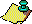Contents Next: Iteration Construction Up: The Symbolic Search Previous: Initial values

The problem is to find a recurrence relation for the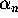, defined in (4.1). An examination of (3.18) reveals that we need to get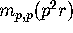in terms of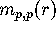. From (3.14) we see that this is equivalent to finding a relation between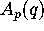,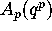and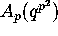. Here we have written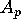as a function of q and not r. By using (3.15), it can be shown thatis a modular form of weight two on a certain congruence subgroup.

This implies that all three functions,andare modular forms of weight two on a certain congruence subgroup and hence must satisfy an algebraic relation of the form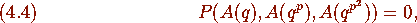where P is a certain rational homogeneous polynomial of degree k, say. The crucial observation is that the left-hand side of (4.4) is a modular form of weight 2k. It is well-known that

the dimension of the space of modular forms (above) of weight 2k is asymptotic to some postive constant times k (see ). It is also well-known that the number of monomials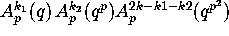is asymptotically equal to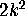. Hence there will always be a relation for large enough k. Such a relation can be found and proved symbolically. This is really a linear problem. The q-series expansion of each monomial up to a certain power of q can be easily computed and stored as a column in a matrix. Finding homogeneous relations is then equivalent to finding the nullspace of a certain matrix. Such relations can be proved by verifying them to a high enough power of q using the theory of modular forms . See  for more details. We illustrate the case p=2 with a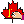MAPLE session.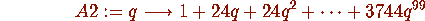> findhom([A2(q),A2(q),A2(q)],2,100);

# of terms, 22

-----RELATIONS-----of order---,2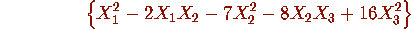We now check the relations to O(qt)

---RELATION----, 1, ---checks to order---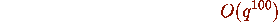The function Aseries(2) gives the q-series expansion of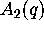. Using our function findhom we found that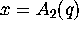,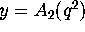,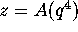seem to satisfy the equation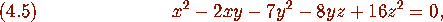at least to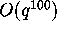, which is enough for a proof. By solving this equation we see that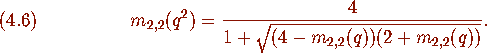Contents Next: Iteration Construction Up: The Symbolic Search Previous: Initial values